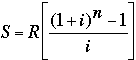SEARCH HOMEMath Central Quandaries & QueriesQuestion from George, a student: To save for his daughter's university education at the end of each month Mr McLean sets aside £50 in a savings scheme paying 10% p.a. compounded monthly. He begins saving when his daughter is 3 years old. How much will he have saved by the time his daughter is 18?George,

If you are expected to use the standard formula to solve this problem then here is the formulawhere S is the value at the end of the time period, R is the amount of the monthly deposit, i is the interest rate per period (for your question i = 0.10/12) and n is the number of periods (number of months).

If you are to answer this from first principles, without the formula, write back.

HarleyMath Central is supported by the University of Regina and The Pacific Institute for the Mathematical Sciences.